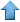Precision RecruitingData Mining Contest Math Jobs * Site Map
 [ Home ] [ Finance ] [ Web Audit ] [ Consulting ]

These are difficult mathematical questions. They are arising from real applications such as fraud detection, arbitrage and scoring systems. If you have interesting answers to any questions, feel free to email us your comments or solution. The best answers will be published here. Companies and Organizations interested in submitting problems should E-mail us.

Scorecards: Logistic, Ridge and Logic Regression

In the context of credit scoring, one tries to develop a predictive model using a regression formula such as Y = Σ wi Ri, where Y is the logarithm of odds ratio (fraud vs. non fraud). In a different but related framework, we are dealing with a logistic regression where Y is binary, e.g. Y = 1 means fraudulent transaction, Y = 0 means non fraudulent. The variables Ri, also referred to as fraud rules, are binary flags, e.g.

• high dollar amount transaction
• high risk country
• high risk merchant category

This is the first order model. The second order model involves cross products Ri x Rj to correct for rule interactions. The purpose of this question is to how best compute the regression coefficients wi, also referred to as rule weights. The issue is that rules substantially overlap, making the regression approach highly unstable. One approach consists of constraining the weights, forcing them to be binary (0/1) or to be of the same sign as the correlation between the associated rule and the dependent variable Y. This approach is related to ridge regression. We are wondering what are the best solutions and software to handle this problem, given the fact that the variables are binary.

Note that when the weights are binary, this is a typical combinatorial optimization problem. When the weights are constrained to be linearly independent over the set of integer numbers, then each Σ wi Ri (sometimes called unscaled score) corresponds to one unique combination of rules. It also uniquely represents a final node of the underlying decision tree defined by the rules.

Contributions:Data Mining • Machine Learning • Analytics • Quant • Statistics • Econometrics • Biostatistics • Web Analytics • Business Intelligence • Risk Management • Operations Research • AI • Predictive Modeling • Actuarial Sciences • Statistical Programming • Customer Insight • Data Modeling • Competitive Intelligence • Market Research • Information Retrieval • Computer Science • Retail Analytics • Healthcare Analytics • ROI Optimization • Design Of Experiments • Scoring Models • Six Sigma • SAS • Splus • SAP • ETL • SPSS • CRM • Cloud Computing • Electrical Engineering • Fraud Detection • Marketing Databases • Data Analysis • Decision Science • Text Mining